# 1350 Tangent Lines

Tangent

## Math 1350 - Differentiation and Tangent Lines

In this lab you will see how to find the derivative of a function and how to find the tangent line. The equation of the tangent line at x = a is

L(x) = f(a)+f_prime(a)*(x-a).

Example1:

differentiate f(x)=2*x^3/5.

> f:=x->2*x^3/5;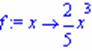To differentiate f(x) use MAPLE command D(f).

> f_prime:=D(f);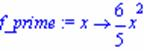Find the value for f_prime for x=5.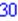Example 2:

Find the tangent line for f(x) = x^2, at a = 2. Plot the function and its tangent line.

First we define the function

> f:=x->x^2;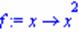To find the slope of the tangent line to the curve y = f(x), differentiate f(x). Then apply the formula for the tangent line.

> f_prime:=D(f);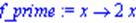> L:=x->f(2)+f_prime(2)*(x-2);> plot([f(x), L(x)], x=-4..4, y=-5..10);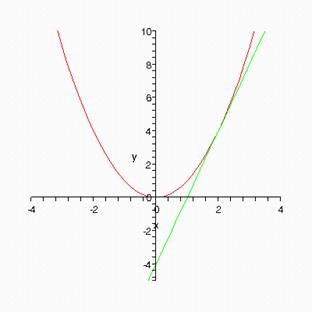Exercises:

1)     Find the tangent line for f(x) = x^4-5*x^3+x^2-1 at a = -2 and a = -4. Plot f(x) and its tangent lines.

2)     Find the tangent line of f(x) = cos(x) at? a = p/2. Plot f(x) and its tangent line.